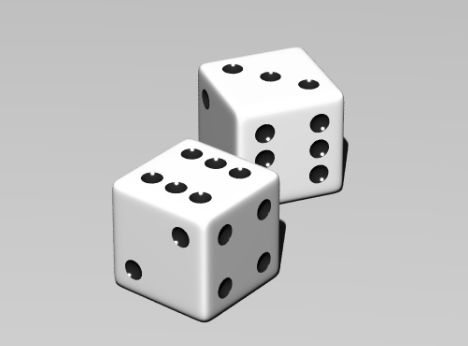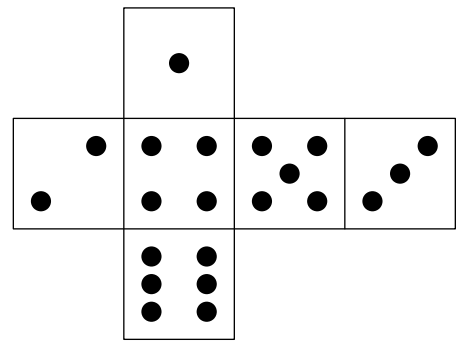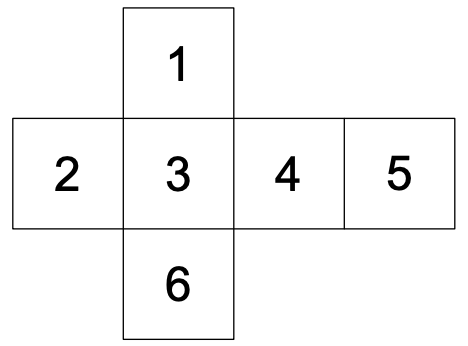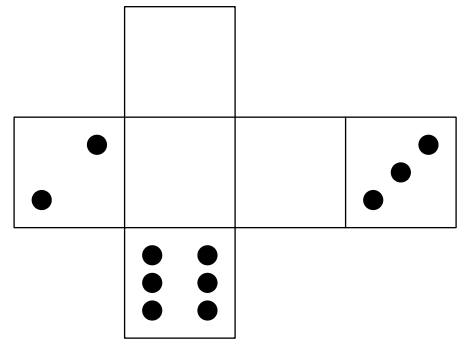시간 제한메모리 제한제출정답맞은 사람정답 비율
2 초 512 MB0000.000%

## 문제

The die is cast.

— Julius Caesar (100 B.C. – 45 B.C.)

Dice have a very long history. It is not clear when and where dice are originated. It is known, however, that dice have been used in Egypt since the time before the year of 3000 B.C.Figure 1: Picture of dice

Dice are sometimes represented by their nets as shown in Figure 2 when they are depicted on a plane. As you know, there are a number of nets that represent the same dice. In this problem, however, we consider only nets of the same shape as the one shown in Figure 2. For our convenience, we index the faces as shown in Figure 3.Figure 2: Net of dieFigure 3: Indices of faces

One day, Dr. M introduced the concept of the optimum nets of dice. He defined the net with the minimum M-value as optimal. The M-value is given by the sum of differences of the numbers on adjacent faces of the net. For Figure 2, the M-value is |1 − 4| + |2 − 4| + |4 − 5| + |5 − 3| + |4 − 6| = 9. Because Dr. M has a little misunderstanding about dice, the sum of the numbers on the opposite faces is not always seven. Nevertheless, each face has a number from one to six, and no two different faces have the same number.

As a result of his research, he discovered the optimum nets under various conditions. However, unfortunately, part of his nets got unable to read because he had spilt coffee over his notebook by mistake.

Your task is to write a program to recover his nets of dice from their parts.Figure 4: Net given as the sample input; unreadable faces indicated by empty.

## 입력

The first line of the input contains one positive integer T indicating the number of test cases. The following T lines contain the test cases.

Each test case consists of only one line that contains six characters, each of which is either a digit from ‘1’ to ‘6’, or a lowercase ‘x’. The i-th character corresponds to the face indexed i in Figure 3. A digit represents the number on the face; a ‘x’ indicates that the number is unreadable, thus must be determined by your program. No test case contains more than one same digit.

## 출력

For each test case, output one line containing six digits that denote the optimum net under the condition that numbers on faces given in the input must be unchanged. The i-th digit must represent the number on the face indexed i in Figure 3, as in the input. If there is more than one solution, output any of them.

## 예제 입력 1

1
x2xx36


## 예제 출력 1

124536